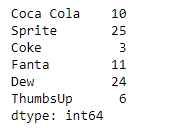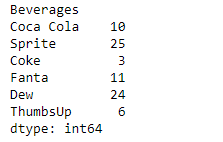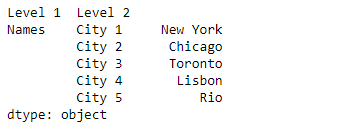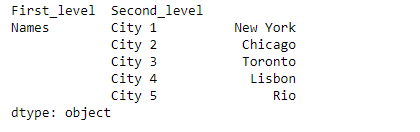Skip to content
Related Articles
Python | Pandas Series.rename_axis()
• Last Updated : 10 Feb, 2019

Pandas series is a One-dimensional ndarray with axis labels. The labels need not be unique but must be a hashable type. The object supports both integer- and label-based indexing and provides a host of methods for performing operations involving the index.

Pandas` Series.rename_axis()` function is used to set the name of the axis for the index or columns.

Syntax: Series.rename_axis(mapper=None, index=None, columns=None, axis=None, copy=True, inplace=False)

Parameter :
mapper : Value to set the axis name attribute.
index, columns : A scalar, list-like, dict-like or functions transformations to apply to that axis’ values.
axis : The axis to rename.
copy : Also copy underlying data.
inplace : Modifies the object directly, instead of creating a new Series or DataFrame.

Returns : Series, DataFrame, or None

Example #1: Use `Series.rename_axis()` function to rename the axis of the given Series object.

 `# importing pandas as pd``import` `pandas as pd`` ` `# Creating the Series``sr ``=` `pd.Series([``10``, ``25``, ``3``, ``11``, ``24``, ``6``])`` ` `# Create the Index``index_ ``=` `[``'Coca Cola'``, ``'Sprite'``, ``'Coke'``, ``'Fanta'``, ``'Dew'``, ``'ThumbsUp'``]`` ` `# set the index``sr.index ``=` `index_`` ` `# Print the series``print``(sr)`

Output :Now we will use `Series.rename_axis()` function to rename the axis of the given series object.

 `# rename the axis``result ``=` `sr.rename_axis(``'Beverages'``)`` ` `# Print the result``print``(result)`

Output :As we can see in the output, the `Series.rename_axis()` function has successfully renamed the axis of the given series object.

Example #2 : Use `Series.rename_axis()` function to rename the MultiIndex axis of the given Series object.

 `# importing pandas as pd``import` `pandas as pd`` ` `# Creating the Series``sr ``=` `pd.Series([``'New York'``, ``'Chicago'``, ``'Toronto'``, ``'Lisbon'``, ``'Rio'``])`` ` `# Create the MultiIndex``index_ ``=` `pd.MultiIndex.from_product([[``'Names'``], [``'City 1'``, ``'City 2'``, ``'City 3'``, ``'City 4'``, ``'City 5'``]],``                                                                     ``names ``=``[``'Level 1'``, ``'Level 2'``])`` ` `# set the index``sr.index ``=` `index_`` ` `# Print the series``print``(sr)`

Output :Now we will use `Series.rename_axis()` function to rename the axis of the given series object.

 `# rename both the levels of the axis of ``# the given series object``result ``=` `sr.rename_axis([``'First_level'``, ``'Second_level'``])`` ` `# Print the result``print``(result)`

Output :As we can see in the output, the `Series.rename_axis()` function has successfully renamed both the levels of the axis of the given series object.

Attention geek! Strengthen your foundations with the Python Programming Foundation Course and learn the basics.

To begin with, your interview preparations Enhance your Data Structures concepts with the Python DS Course. And to begin with your Machine Learning Journey, join the Machine Learning – Basic Level Course

My Personal Notes arrow_drop_up